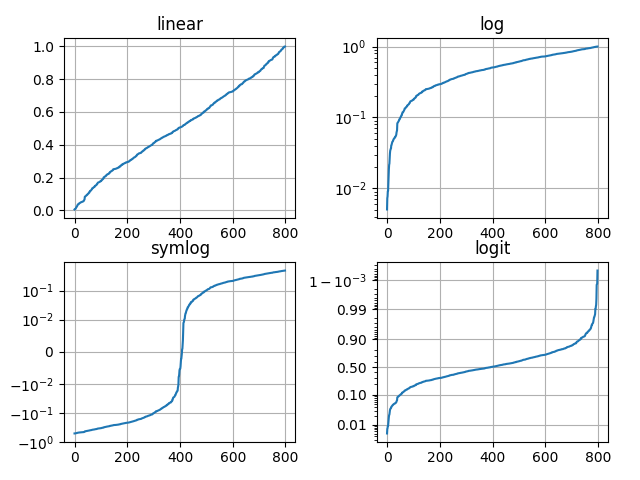# [译]Pyplot教程¶

Pyplot接口简介

## Pyplot介绍¶

matplotlib.pyplot是命令样式函数的集合，目的是让matplotlibMATLAB一样工作。每个pyplot函数都对图像做一些改变：比如，创建一张图像、在图形中创建绘图区域、在绘图区域中绘制一些线条、用标签装饰绘图，等等

matplotlib.pyplot中，不同的状态在函数调用之间被保留，这样它就可以跟踪当前图形和绘图区域，并且绘图功能被定向到当前轴（请注意，这里的“轴”以及文档中大多数地方指的是绘图区域，参考下图，而不是严格意义上的数学术语）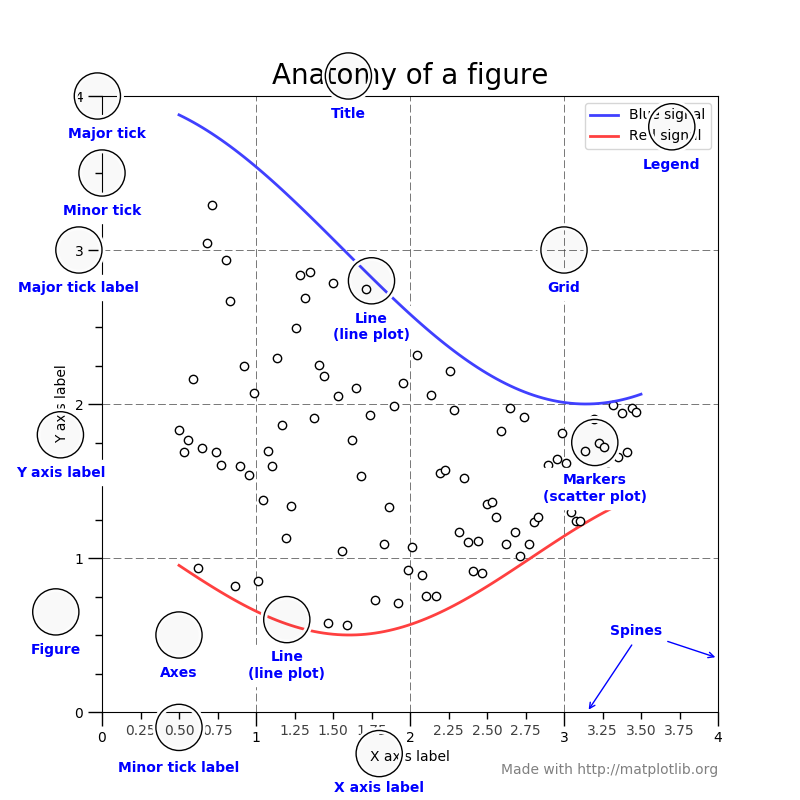import matplotlib.pyplot as plt
plt.plot([1, 2, 3, 4])
plt.ylabel('some numbers')
plt.show()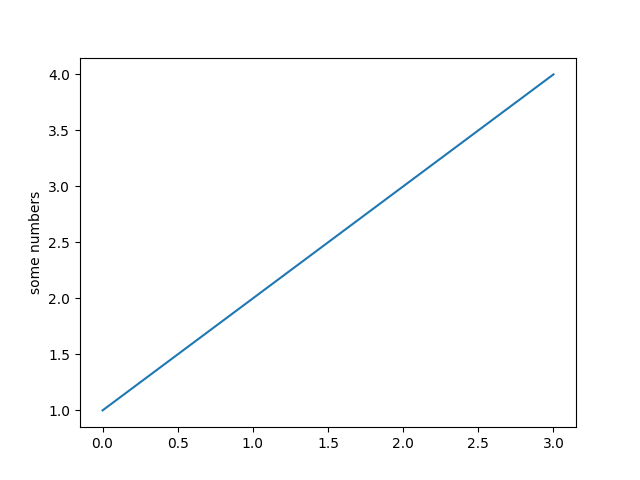plot()是一个通用的命令，可以接受任意数量的参数。例如，要绘制xy，可以发出以下命令：

plt.plot([1, 2, 3, 4], [1, 4, 9, 16])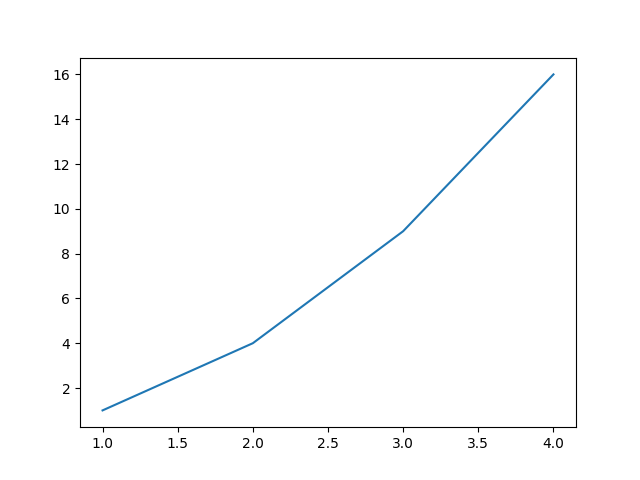## 格式化绘图样式¶

plt.plot([1, 2, 3, 4], [1, 4, 9, 16], 'ro')
plt.axis([0, 6, 0, 20])
plt.show()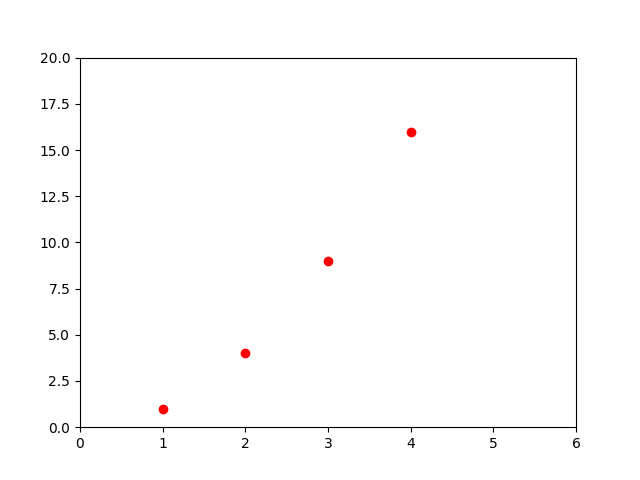import numpy as np

# evenly sampled time at 200ms intervals
t = np.arange(0., 5., 0.2)

# red dashes, blue squares and green triangles
plt.plot(t, t, 'r--', t, t**2, 'bs', t, t**3, 'g^')
plt.show()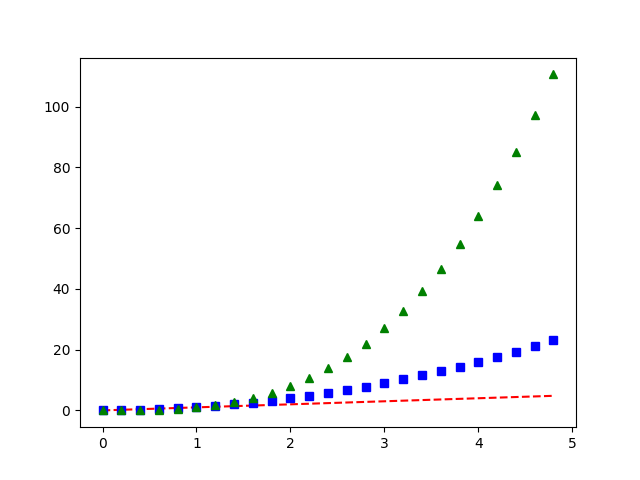## 使用关键字字符串绘图¶

matplotlib允许您为此类对象提供data关键字参数。如果提供，则可以使用与这些变量对应的字符串生成绘图

data = {'a': np.arange(50),
'c': np.random.randint(0, 50, 50),
'd': np.random.randn(50)}
data['b'] = data['a'] + 10 * np.random.randn(50)
data['d'] = np.abs(data['d']) * 100

plt.scatter('a', 'b', c='c', s='d', data=data)
plt.xlabel('entry a')
plt.ylabel('entry b')
plt.show()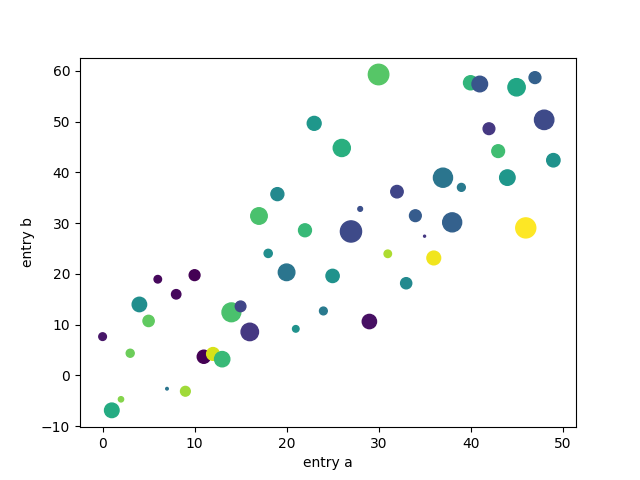## 使用分类变量绘图¶

names = ['group_a', 'group_b', 'group_c']
values = [1, 10, 100]

plt.figure(1, figsize=(9, 3))

plt.subplot(131)
plt.bar(names, values)
plt.subplot(132)
plt.scatter(names, values)
plt.subplot(133)
plt.plot(names, values)
plt.suptitle('Categorical Plotting')
plt.show()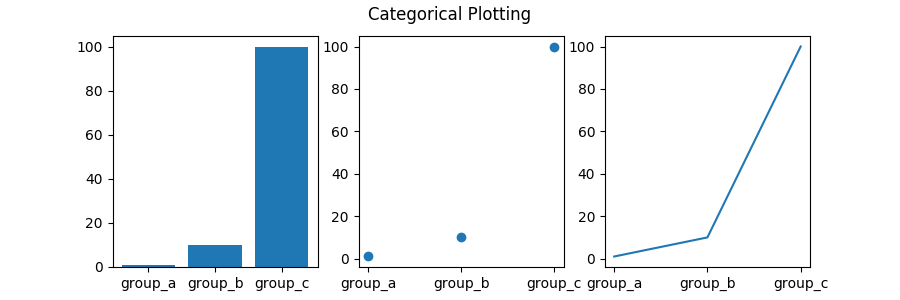## 控制线条属性¶

• 使用关键字参数
plt.plot(x, y, linewidth=2.0)

• 使用Line2D实例的setter方法。plot返回一列Line2D对象，比如line1, line2 = plot(x1, y1, x2, y2)。在下面的代码中，我们假设只有一行，因此返回的列表长度为1。我们使用元组解压的方式来获取该列表的第一个元素：
line, = plt.plot(x, y, '-')
line.set_antialiased(False) # turn off antialising

• 使用setp()属性。下面示例使用matlab样式的命令在一个行列表上设置多个属性。setp透明地处理对象列表或单个对象。您可以使用python关键字参数或matlab样式的字符串/值对：
lines = plt.plot(x1, y1, x2, y2)
# use keyword args
plt.setp(lines, color='r', linewidth=2.0)
# or MATLAB style string value pairs
plt.setp(lines, 'color', 'r', 'linewidth', 2.0)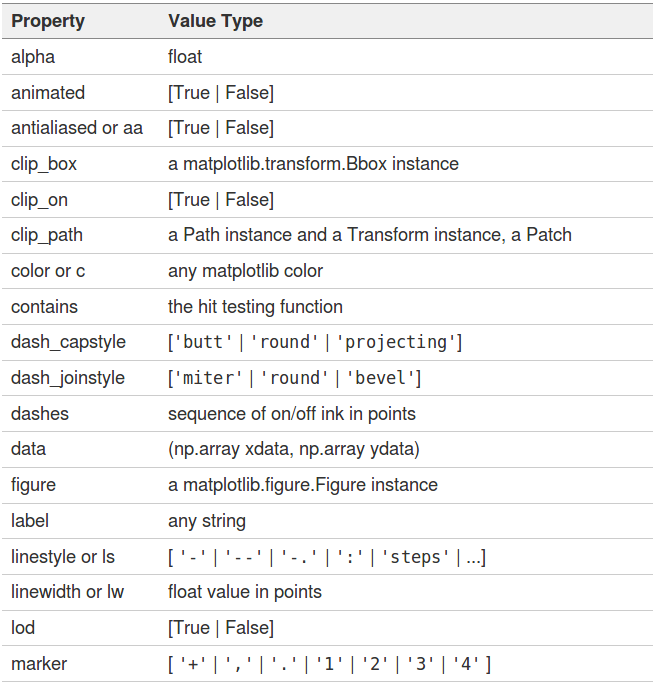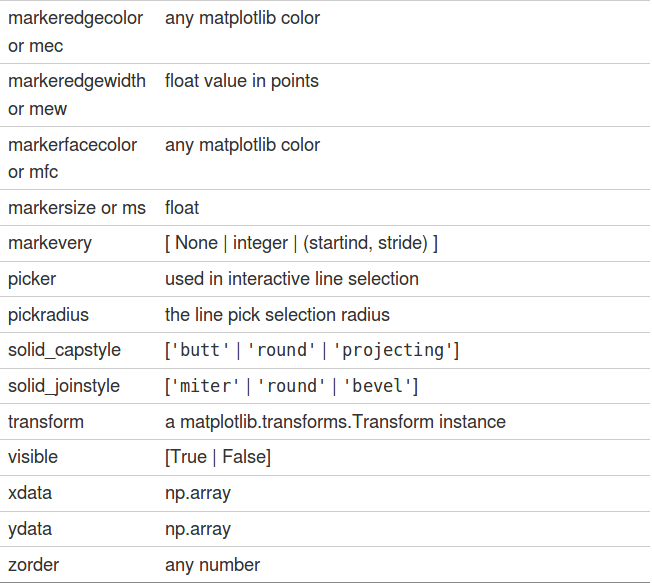>>> import matplotlib.pyplot as plt
>>> lines = plt.plot([1, 2, 3])
>>> plt.setp(lines)
agg_filter: a filter function, which takes a (m, n, 3) float array and a dpi value, and returns a (m, n, 3) array
alpha: float
animated: bool
...
...


## 使用多个图形和轴¶

matlabpyplot都有当前图形和当前轴的概念。所有绘图命令都应用于当前轴。函数gca()返回当前轴（matplotlib.axes.Axes实例），gcf()返回当前图形（matplotlib.figure.figure实例）。通常情况下，你不必担心这件事，因为它都是在幕后处理的。下面是创建两个子图的脚本

def f(t):
return np.exp(-t) * np.cos(2*np.pi*t)

t1 = np.arange(0.0, 5.0, 0.1)
t2 = np.arange(0.0, 5.0, 0.02)

plt.figure(1)
plt.subplot(211)
plt.plot(t1, f(t1), 'bo', t2, f(t2), 'k')

plt.subplot(212)
plt.plot(t2, np.cos(2*np.pi*t2), 'r--')
plt.show()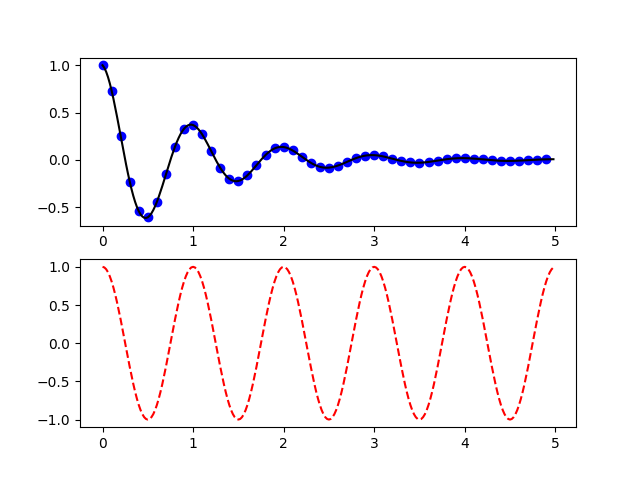import matplotlib.pyplot as plt
plt.figure(1)                # the first figure
plt.subplot(211)             # the first subplot in the first figure
plt.plot([1, 2, 3])
plt.subplot(212)             # the second subplot in the first figure
plt.plot([4, 5, 6])

plt.figure(2)                # a second figure
plt.plot([4, 5, 6])          # creates a subplot(111) by default

plt.figure(3)                # figure 1 current; subplot(212) still current
plt.subplot(211)             # make subplot(211) in figure1 current
plt.title('Easy as 1, 2, 3') # subplot 211 title

plt.show()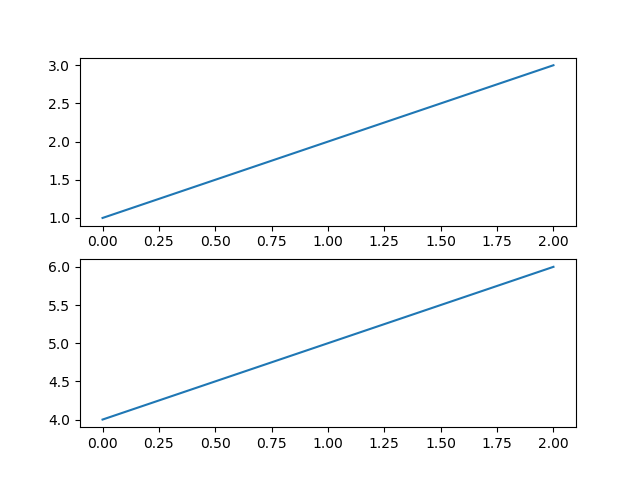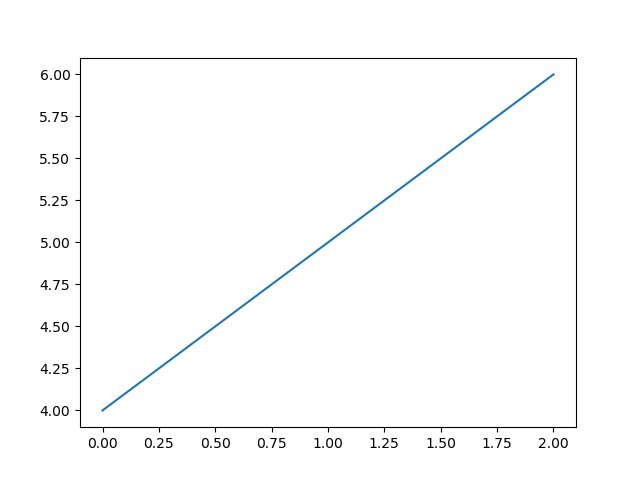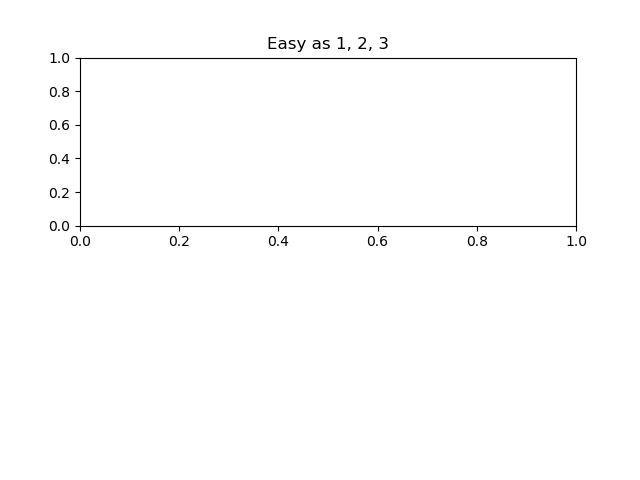## 文本工作¶

text()命令可用于在任意位置添加文本，xlable()ylabel()title()用于在指定位置添加文本。

mu, sigma = 100, 15
x = mu + sigma * np.random.randn(10000)

# the histogram of the data
n, bins, patches = plt.hist(x, 50, density=1, facecolor='g', alpha=0.75)

plt.xlabel('Smarts')
plt.ylabel('Probability')
plt.title('Histogram of IQ')
plt.text(60, .025, r'$\mu=100,\ \sigma=15$')
plt.axis([40, 160, 0, 0.03])
plt.grid(True)
plt.show()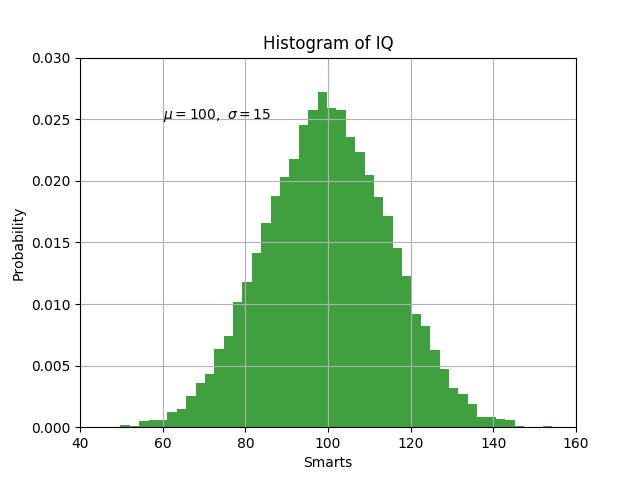t = plt.xlabel('my data', fontsize=14, color='red')


## 在文本中使用数学表达式¶

matplotlib接受任何文本表达式中的tex表达式。比如在标题中写入$\sigma_{i}=15$，可以写一个TeX表达式，用$符号括起来： plt.title(r'$\sigma_i=15\$')


## 注解文本¶

ax = plt.subplot(111)

t = np.arange(0.0, 5.0, 0.01)
s = np.cos(2*np.pi*t)
line, = plt.plot(t, s, lw=2)

plt.annotate('local max', xy=(2, 1), xytext=(3, 1.5),
arrowprops=dict(facecolor='black', shrink=0.05),
)

plt.ylim(-2, 2)
plt.show()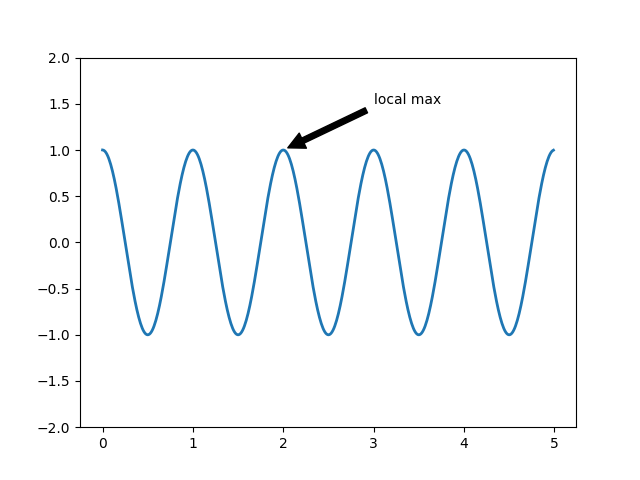## 对数轴和其他非线性轴¶

pyplot不仅支持线性轴比例，还支持对数和逻辑比例。如果数据跨越多个数量级，则通常使用这种方法。更改轴的比例很容易：

plt.xscale('log')


from matplotlib.ticker import NullFormatter  # useful for logit scale

# Fixing random state for reproducibility
np.random.seed(19680801)

# make up some data in the interval ]0, 1[
y = np.random.normal(loc=0.5, scale=0.4, size=1000)
y = y[(y > 0) & (y < 1)]
y.sort()
x = np.arange(len(y))

# plot with various axes scales
plt.figure(1)

# linear
plt.subplot(221)
plt.plot(x, y)
plt.yscale('linear')
plt.title('linear')
plt.grid(True)

# log
plt.subplot(222)
plt.plot(x, y)
plt.yscale('log')
plt.title('log')
plt.grid(True)

# symmetric log
plt.subplot(223)
plt.plot(x, y - y.mean())
plt.yscale('symlog', linthreshy=0.01)
plt.title('symlog')
plt.grid(True)

# logit
plt.subplot(224)
plt.plot(x, y)
plt.yscale('logit')
plt.title('logit')
plt.grid(True)
# Format the minor tick labels of the y-axis into empty strings with
# NullFormatter, to avoid cumbering the axis with too many labels.
plt.gca().yaxis.set_minor_formatter(NullFormatter())
# Adjust the subplot layout, because the logit one may take more space
# than usual, due to y-tick labels like "1 - 10^{-3}"
plt.subplots_adjust(top=0.92, bottom=0.08, left=0.10, right=0.95, hspace=0.25,
wspace=0.35)

plt.show()